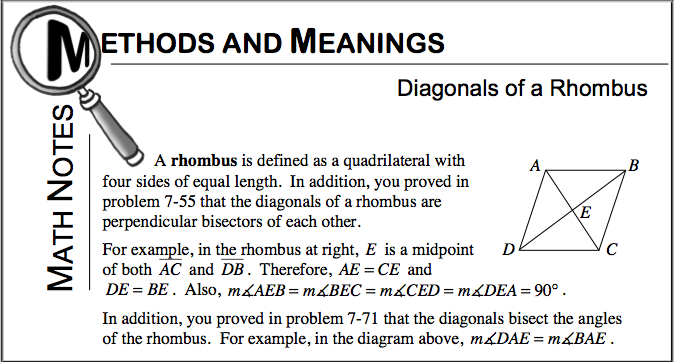Home > CCG > Chapter 7 > Lesson 7.2.4 > Problem7-87

7-87.

The diagonals of a rhombus are $6$ units and $8$ units long. What is the area of the rhombus? Draw a diagram and show all reasoning. Homework Help ✎

Refer to the Math Notes box below from Lesson 7.2.4.To ''bisect'' means to divide into two equal parts. Perpendicular objects form $90°$ angles.